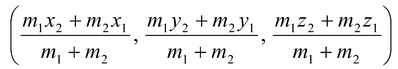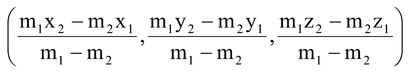# Section formulas

## Section formulas

### Section Formula for Internal Division

Let P(x1, y1, z1) and Q(x2, y2, z2) be two points.

Let R be a point on the line segment joining P and Q such that it divides the join of P and Q internally in the ratio m1 : m2. Then the coordinates of R are### Section Formula For External Division

Let P(x1, y1, z2) and Q(x2, y2, z2) be two points, and let R be a point on PQ produced dividing it externally in the ratio m1 : m2 (m1 ≠ m2). Then the coordinates of R are